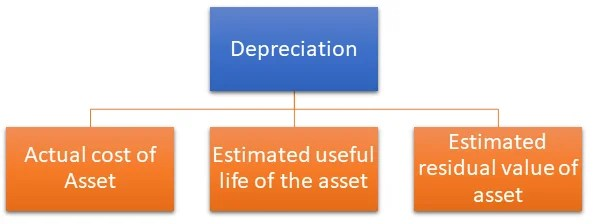# Difference between Depreciation, Amortization and Depletion

26th November 2020

Depreciation

Depreciation is the accounting term used for assets such as buildings, furniture and fittings, equipment etc. Companies use this to record the diminishing value of their assets as they are used in the business from the time of purchase of such assets. Hence cost is allocated periodically as value lost due to usage (as expense affecting the business’s net income) and the declining value of assets is recorded (affecting the value of business). Different methods exist in calculating the depreciation amount and these are different depending on the asset type. The depreciation is calculated from the time an asset is used / placed for service and the depreciation is recorded periodically. Depreciation is calculated taking the cost of the asset, the expected useful life of the asset, residual value of the asset and percentage where necessary. Depreciation is not taken into account once the full cost of the asset is recovered / the asset is no longer in the company’s possession (i.e. sold, stolen and fully depreciated). Two main ways exist in calculating depreciation and they are the straight line (which allows deducting the same amount each year over the life of the asset) and reducing balance method / declining balance method (which provides for a higher charge in the first year and reducing amount throughout the asset life).

Depletion

Depletion is an accounting concept which is used mostly in mining, timber, petroleum or other similar industries. Being similar to depreciation, depletion allows accounting for the reduction of the resource’s reserve. There are two main types of depletion calculation: cost depletion (where cost of the resource allocated over the period) and percentage depletion (the percentage of the property’s gross income where percentage is specified for each mineral).

When dealing with a natural resource also referred as a mineral asset the concept of depreciation or amortization cannot be applied. “Depletion” is a form of a systematic reduction in the value of a natural resource based on the rate at which it is being used.For example: A coal mine has 10 Million tonnes of coal and the coal extraction is happening at the rate of 1 Million tonnes per year. In this case, depletion rate would be 10% p.a. since at this rate of extraction the coal mine is being depleted at 10% per year.

Though both have similar concepts, difference between depreciation and depletion exist as mentioned below.

1. Depreciation is on tangible assets whereas depletion is on non-renewable resources.
2. Depreciation is the deduction of the asset value due to aging, whereas depletion is the actual physical reduction of the company’s natural resources (accounting for consumption).

Amortization

Prorating cost of an “Intangible Asset” over the period during which benefits of this asset are estimated to last is called Amortization. The concept of amortization is also used with leases & debt repayment.

Amortization is for Intangible assets whereas depreciation is for tangible fixed assets. Examples of intangible assets are copyrights, patents, software, goodwill, etc.

 Method of Reduction Type of Asset Examples Depreciation Fixed Assets Building, Machinery etc. Amortization Intangible Assets Copyright, Patent etc. Depletion Mineral Assets Mines, Oil fields etc.